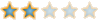Montreal Math ClubMathematics students in Montreal - Étudiants de mathématiques à MontréalHomeCalendarFAQSearchMemberlistUsergroupsRegisterLog in

 Montreal Math Club :: Chit chat :: The Water Cooler Share

Duality - is it necessary ?AuthorMessage

EuclidPosts : 95
Join date : 2009-09-15
Age : 57
Location : AlexandriaSubject: Duality - is it necessary ?Sat Oct 17, 2009 4:53 pm One way or another the duality occurs in math.For example, let me define the dual of a pair (a, b) as (b, a) with the notation(a, b)* := (b, a) Of course,(a, b)** = (b, a)* = (a, b). But this duality is more touchy : for example, we can prove diff( (f, g)*) = (diff(f, g)) * and so on. Another example of duality : Let A = { 1,2,3, 4 } be a set of individuals x and f, g, h one-to-one maps on A onto K = {a, b, c, d,}- | f, g, h 1 | a b c 2 | b c a3 | c d b4 | d a dWe have f(1)= a, f(2)= b,..., h(4) = d. There is a risk that someone that does not know the 'right' notations to see the table as functions defined on { f, g, h } :1(f) = a, 1(g) = b,... 4(h) = d; so, x(f) = f(x).Thus, to maintain the coherence of 'right' notations, we write x*(F) instead of x(F) to say the same thing. Of course, x**(F) = (x*(F))* = (F(x))*= F*(x) = x(F) for every F and every x.Now comes my question : Has anyone an example where the duality brings more than a rewriting of something, when it is necessary to a result that can't be proved else ?The first example could be the contrapositive : contrapositive (contrapositive (implication)) = implicationTo prove that sqrt(2) is not rational, one needs to prove the contrapositive.Duality - is it necessary ?Page 1 of 1

Permissions in this forum:You cannot reply to topics in this forumJump to: Select a forum||--Math Club|   |--Mathematical Sundays|   |--Suggestions|   |--Introduce yourself|   |--Mathematics|   |--Problem Solving|   |   |--Solved problems|   |   |   |--Calculus and Analysis|   |--Combinatorics|   |--Geometry|   |--Number Theory|   |--Probabilities and statistics|   |--Set theory and Topology|   |--Online Resources|   |--Chit chat    |--The Water Cooler    |--Mathematical poems